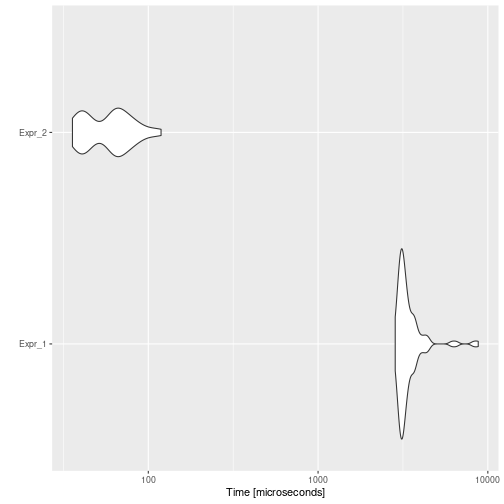# Dead Code Elimination

## Background

Dead Code Elimination is an optimization that removes code which does not affect the program results. You might wonder why someone would write this type of source code, but it can easily creep into large, long-lived programs even at the source code level. Removing such code has several benefits: it shrinks program size and it allows the running program to avoid executing irrelevant operations, which reduces its running time. It can also enable further optimizations by simplifying program structure.

For example, consider the following code:

``````foo <- function() {
a <- 24
if (a > 25) {
return(25)
a <- 25 # dead code
}
return(a)
b <- 24 # dead code
return(b) # dead code
}
``````

In functions, after calling `return`, the following code would not be executed, so it is dead code and can be eliminated. In this example, resulting in:

``````foo <- function() {
a <- 24
if (a > 25) {
return(25)
}
return(a)
}
``````

Also, after constant propagating and folding we would get:

``````foo <- function() {
a <- 24
if (FALSE) { # dead code
return(25) # dead code
} # dead code
return(a)
}
``````

So it could be reduced to:

``````foo <- function() {
a <- 24
return(a)
}
``````

This dead code optimizer also removes code after `next` or `break` calls.

## Example

Consider the following example:

``````code <- paste(
"i <- 0",
"n <- 1000",
"while (i < n) {",
"  if (TRUE) {",
"    i <- i + 1",
"  } else {",
"    i <- i - 1",
"  }",
"}",
sep = "\n"
)
cat(code)
``````
``````## i <- 0
## n <- 1000
## while (i < n) {
##   if (TRUE) {
##     i <- i + 1
##   } else {
##     i <- i - 1
##   }
## }
``````

Then, the automatically optimized code would be:

``````opt_code <- opt_dead_code(list(code))
cat(opt_code\$codes[])
``````
``````## i <- 0
## n <- 1000
## while (i < n) {
##   i <- i + 1
## }
``````

And if we measure the execution time of each one, and the speed-up:

``````bmark_res <- microbenchmark({
eval(parse(text = code))
}, {
eval(parse(text = opt_code))
})
autoplot(bmark_res)
````````````speed_up(bmark_res)
``````
``````##            Min.  1st Qu.   Median     Mean 3rd Qu.     Max.
## Expr_2 79.66826 72.47271 51.61431 57.04419 48.6184 73.84267
``````

## Implementation

The `opt_dead_code` optimizer performs two main tasks:

### Remove code after interruption commands

All the code, that is equally-nested, found after a `break`, `next`, or `return` call is removed. Something important to note is that it assumes that the `return` function has not been overwritten.

### Remove constant conditionals

This task has sub-items:

• Remove `FALSE` whiles: `while (FALSE) { expr }` expressions are removed from the code.

• Remove `FALSE` ifs: `if (FALSE) { expr }` expressions are removed. And `if (FALSE) { expr1 } else { expr2 }` is replaced by `expr2`.

• Replace `TRUE` ifs: `if (TRUE) { expr }` is replaced by `expr`. And `if (TRUE) { expr1 } else { expr2 }` is replaced by `expr1`.